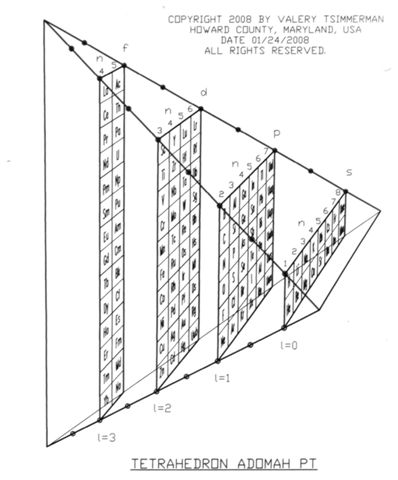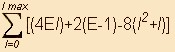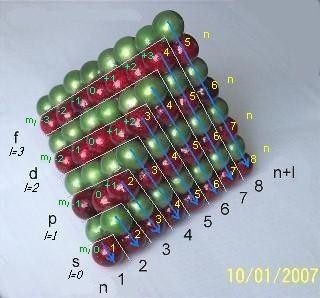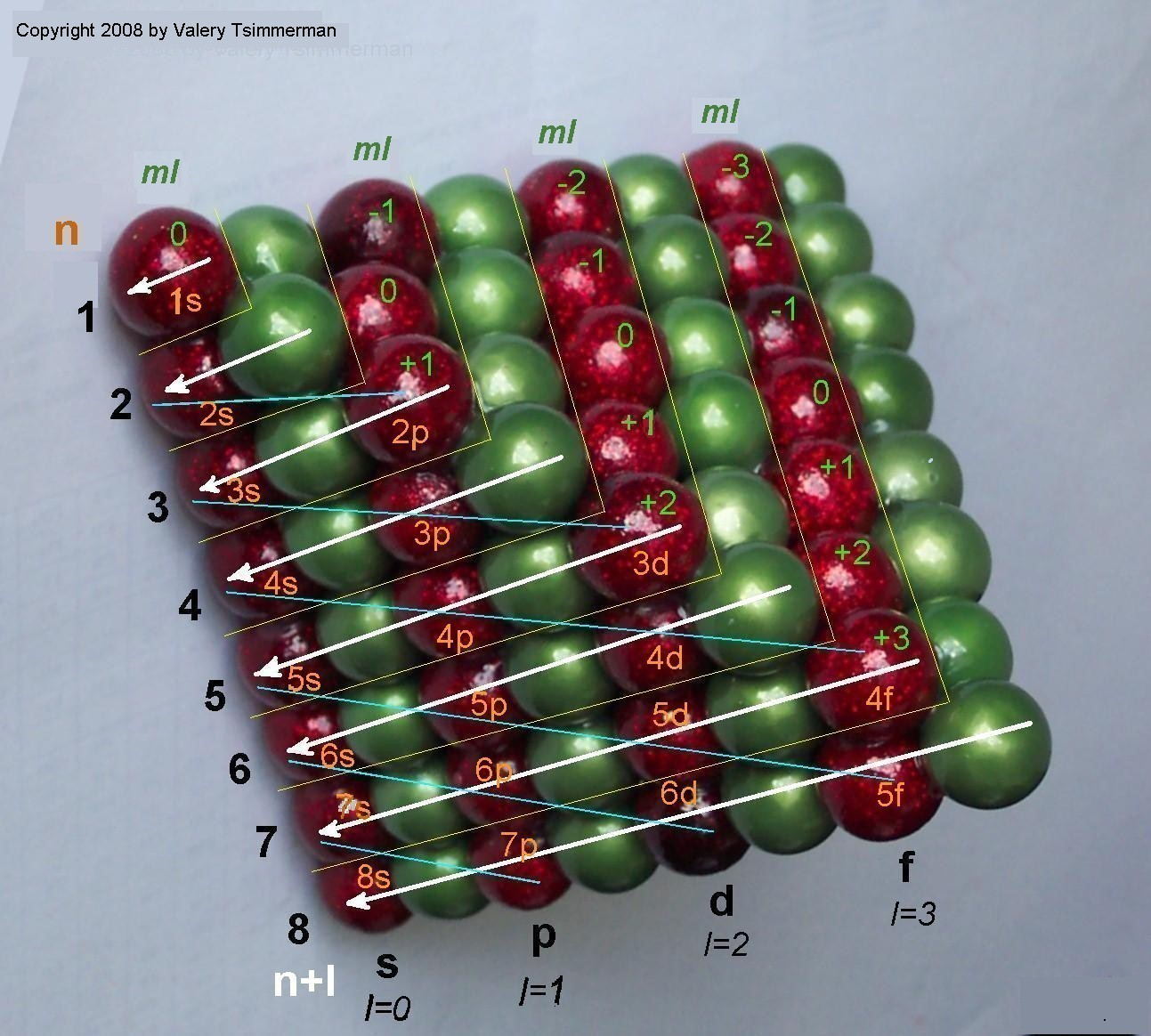Home title

### << Home

####On Tetrahedral Character of the Periodic System.

ADOMAH Periodic Table is built strictly in accordance with the quantum numbers n, l, ml and ms. It comprises four rectangular blocks: s, p, d and f, containing 16, 36, 40 and 28 elements respectively and it closely follows electron configurations of atoms.

Surprisingly, when elements were placed in rectangular boxes, instead of traditional square boxes, perimeters of s,p,d and f blocks became equal!

(refer to "Description" page for more discussion on proportions of the blocks).

Since electrons tend to form pairsand each element corresponds to one characteristic electron, it is logical to place elements in 1/2x1 rectangular cells, so two such cells would form 1x1 unit. This adjustment resulted in block perimeters equal to 18 units:

s-block is 1 unit high and 8 units long (or wide) (1+8 = 9, is half of the perimeter);

p-block is 3 units high and 6 units long (3+6 = 9);

d-block is 5 units high and 4 units long (5+4 = 9);

f-block is 7 units high and 2 units long (7 +2 = 9);

Therefore, perimeter of each block: P=2x9=18 units.

Those are the only four possible rectangles that could have perimeter of 18 (if only natural numbers are used). What can it possibly mean? Could it be just a coincidence?

Apparently not, this is not a coincidence. There is one 3D geometric shape that, if sliced in a certain way, would produce rectangles with the same proportions, orientation, alignment and order as spdf blocks of ADOMAH Periodic Table. This shape is Regular Tetrahedron.

If a regular tetrahedron with edge E is intersected by a plane that is parallel to two opposite edges, cross section will always be a rectangle or a square with perimeter P=2E.

Proportions of fdps blocks of ADOMAH PT, as well as their sequence, alignment and orientation, suggest only one possible conclusion: all four blocks of the Periodic Table are consecutive slices of Regular Tetrahedron, with edges equal to 9 units, produced by the planes spaced 2 units apart, as measured along the edges.

There is another indication that the Periodic System has something to do with regular tetrahedron: Atomic Number of every other alkaline earth element (Be, Ca, Ba, Ubn...) corresponds to every second Tetrahedral Number: 1, 4, 10, 20, 35, 56, 84, 120... Remaining alkaline earth elements (Mg, Sr and Ra) have atomic numbers that are equidistant between the Tetrahedral Numbers shown above in bold: 12=(4+20)/2, 38=(20+56)/2, 88=(56+120)/2. Alkaline earths form the base of ADOMAH Periodic Table. See more on this topic below.

Generally, a Periodic System of any size can be described in terms of a regular tetrahedron with edge E:

1) number of the periods NOP=E-1;

2) number of values of the quantum number 'n': n max=E-1

3) number of blocks representing subshells (number of values of 'l'): NOS=ceil(0.5(E-1)), where ceil stands for "ceiling function", that means "rounded to a higher integer if the result has fractional part" (in case of even 'E').

4) maximum value of quantum number 'l': l max=ceil(0.5(E-3))

5) dimensions of the subshell blocks corresponding to l =0,1..., l max:

as measured along the periods a=4l +2 elements; as measured along the groups b=E-(2l +1).

6) number of elements in each block corresponding to quantum number l =0,1..., l max:

N=(4El)+2(E-1)-8(l2+l)

7) length of the periods: LP=2(l' +1)2 ( here, l' is the maximum value of l for each period).

8) number of the elements (NOE) in the periodic system that corresponds to the tetrahedron with edge 'E':, where lmax=ceil(0.5(E-3)) as defined above.

Notes:

1. Only natural numbers shall be used for the variables (minimum value of 'E' is 2, that is E=2, 3, 4...); 2. All fractional results shall be rounded to a higher integer; 3. Current periodic table (up to 120 elements) is defined by the regular tetrahedron with the edge E=9. The next size of the tetrahedron (E=10) would describe the periodic system with up to 170 elements.

The formulae above clearly demonstrate that all major parameters of a Periodic System can be determined if the edge of the regular tetrahedron 'E', describing such system, is known.Lets make one more step. Instead of presenting each pair of elements as unit square, we will present them as unit spheres and convert the ADOMAH Tetrahedron into the stack of equal spheres, where the layers of the red spheres represent spdf blocks and each individual red sphere represents a pair of elements.

The meaning of the quantum numbers in terms of the tetrahedron stack of spheres would be as follows:

1. Quantum number 'n' corresponds to number of planes normal to the "red edge" of the tetrahedron, which intersect the red spheres only;

2. Quantum number 'l' corresponds to the rectangular layers of the red spheres in the tetrahedral stack shown, including the s-block at the base of the tetrahedron that corresponds to zero 'l' value.

3. Quantum number 'ml' is a deviation of the centers of the rows of the red spheres from the plane of symmetry of the tetrahedron that "cuts" through the s-block and is normal to the "green" edge;

4. Quantum number 'ms' points either to duality of each red sphere or, perhaps, to a double tetrahedron structure.

Now we are ready to demonstrate that ADOMAH Tetrahedron is not just a curious object, but could be an extremely useful tool of the quantum theory.

Currently, the primary quantum number "n" is defined as the sequence: n = 1, 2, 3, 4..., and the quantum number "l" is defined as 0 < l < (n-1), that is: l = 0, ..., (lmax-2), (lmax-1), lmax., where lmax = n-1. However, the old postulate is too simplistic. Interrelation between the quantum number "n" and the quantum number "l" is more complex than that. That is exactly the reason why there are no elements with the ground state electrons in subshell "g" (corresponding to n=5 and l=4) in the current Periodic System. Below are the formulae which describe the interrelation between "n" and "lmax" correctly:

9) if n < E/2, lmax = n-1;

9A) if n > E/2, lmax = E-n-1;

where "E" is the edge of the regular tetrahedron that describes Periodic System (currently E=9 for the system with up to 120 elements). Therefore, if n=5, lmax = 9-5-1 = 3, not 4, as suggested by the old formula.

Formulae (9) and (9A) shown above can be combined in one:

10) lmax = (|(ceil(|n-E/2|+1)-ceil(E/2))| : E=(2,3,4...), n=(1,2,3, ...), n < E), where E as defined above.

This formula agrees with the empirical data better than the old lmax = n-1. It naturally embodies the "n+l" rule, that eludes the explanation via the Schroedinger equation and, despite few attempts to explain it on the basis of energy, the root cause for the filling of the orbitals by the electrons in the order of "n+l", instead of "n", remained a mystery. Madelung rule combines quantization of energy via the quantum number "n" and the quantization of the magnitude of the orbital angular momentum, associated with the quantum number "l".So far, no plausible explanation of "n+l" rule, based on the first principles, has been offered by the physicists. This statement is supported by the fact that the second part of the equation has been missing: n+l = ?

However, this basic rule of the Periodic Law can be explained in terms of the tetrahedral sphere packing. Looking at the tetrahedron stack above and to your right, one can easily notice that 'n+l' numbers shown there represent the consecutive triangular layers of spheres, which begin with the single red sphere that stands for the first two elements H and He and end with 8th layer of the spheres representing elements Ac through Ubn. Aufbauprinzip (or order of filling orbitals) is clearly seen as the tetrahedron builds up, for example:

layer n+l=2 containing elements Li and Be is represented by two green and one red spheres (where the red sphere represents the pair of the elements and the green spheres are the part of the matrix);

layer n+l=3 containing elements B through Mg is represented by three red-p two green and one red-s spheres;

layer n+l=4 containing Al trough Ca is represented by four green, three red-p, two green and one red-s spheres, etc.

Let us find the missing part of the equation that describes the Madelung Rule. From the formula (9A) (see above) that defines the maximum value of the quantum number "l":

lmax = E-n-1, therefore: n+l = E-1, where E = 2, 3, 4... represents the edge of the growing tetrahedron that reflects Bohr's Aufbau Process.

Also, from the formula No.2 presented on this page above: E = n max + 1. Therefore, n+l = n max, where "n max" is the maximum value of the primary quantum number "n" for each triangular layer of spheres of the growing tetrahedral stack defined as "n+l". This explains why the numbers shown at the bottom of the ADOMAH Periodic Table represent both "n" and "n+l".

Let's try the new formula n+l = E-1, to see if it works. Let's assume E=7, current value of the edge of the expanding tetrahedron that represents first 56 elements in the Periodic Table (see formula No.8 above). From the above formula No.2: n max=7-1=6, then, from the formula No.4: l max=0.5(7-3)=2. Therefore, there are only three possible solutions for n+l=7-1:

4+2=6, 5+1=6 and 6+0=6. These combinations represent the red spheres in 6th triangular layer of the stack shown above, or 6th period in Janet's Left Step and the ADOMAH Periodic Tables. It works!

### Successful application of the tetrahedral packing of spheres for the illustration of "n+l" rule, as well as the above formulae (9A) and (10), indicate that there is an additional, previously overlooked, boundary condition to solutions of the Schroedinger equation.To your left is 3D mnemonic diagram that shows the order of filling of orbitals. Third dimension, representing the magnetic quantum number "ml", has become visible in addition to the quantum numbers "n" and "l" which have been traditionally used in two dimensional mnemonics. This is how clearly the close sphere packing illustrates the aufbau principle, the Madelung rule, the quantum numbers and their interrelation.

Looking at the ADOMAH Tetrahedron Stack we can also see why double periodicity exists. Instead of looking at the dull numbers of the quantum mechanics n, l and ml , now we have the way to see them within the geometric structure! It allows us to visualize how quantum numbers are related to each other.

The tetrahedron does not have to stop at n+l=8 layer. If elements 122,124,126... discovered, new n+l=9 layer would be started. However, there are reasons to believe that no elements beyond 120 will be found.

The Hund's rule, that states that the electrons fill as many different orbitals of equal energies as possible, means that the full row of red spheres corresponding to a particular shell n = 1, 2, 3... and block (s, p, d or f) has to be filled with single electrons first, before the doubling begins.

The stack of spheres shown above also explains why number of electrons in atomic shells corresponding to n= 1, 2, 3, 4, 5, 6, 7, 8 are 2,8,18,32,32,18,8,2 and not 2,8,18,32,50,72,98,128, as current quantum theory suggests. The reason for the absence of the elements with ground state electrons in subshells g, h, i and j corresponding to n= 5, 6, 7 and 8 is obvious: The Periodic Law follows the order of the tetrahedral sphere packing.

Here is the new general rule of the Periodic Law that is in complete agreement with the experimental data and, unlike the Madelung rule, has no exceptions:

### The order of orbital filling is such that no ground state electron of an element with atomic number Z<NOE can violate boundaries for the quantum numbers "n", "l" and "ml" defined by the imaginary regular tetrahedron, known as the ADOMAH Tetrahedron, with edge E.

where NOE is the number of elements as defined by the formula (8) shown above and E=(2, 3, 4...) is the edge of the expanding regular tetrahedron that represents the filling of orbitals.

Adomah Tetrahedron Stack of Spheres also solves another scientific mystery: length of periods in Periodic Table versus number of electrons in atomic shells. Only first three periods of the traditional IUPAC Periodic Table follow principal quantum number 'n' (1st period: 1s; 2nd period: 2s, 2p; 3rd period: 3s, 3p). Beginning with the forth period pattern breaks down and periods begin to include elements with the electrons in sub shells that do not correspond to the current 'n' value (4th period: 4s, 3d, 4p; 5th period: 5s, 4d, 5p; 6th period: 6s, 4f, 5d, 6p; etc.) ADOMAH Tetrahedron clarifies this situation:

Number of the electrons in atomic shells that correspond to the quantum number 'n' are equal to twice the number of the red spheres in the planes normal to the edge 's'. On the other hand, number of the elements in the periods that follow 'n+l' rule are equal to twice the number of the red spheres in the planes parallel to the face of the tetrahedron (n+l triangular layers shown above). This clearly demonstrates that, although the sub shells associated with a period in the periodic table have different shell numbers 'n', the length of the periods (2,2,8,8,18,18,32,32) in the Periodic Table and the number of electrons in atomic shells (2,8,18,32,32,18,8,2) is not mere a coincidence, as some scientists believe, but direct consequence of the tetrahedral sphere packing as demonstrated by the ADOMAH Tetrahedron Stack. In other words, those are the two different ways to slice the same tetrahedron.

It was mentioned above that Atomic Number of every other alkaline earth element (Be, Ca, Ba, Ubn...) corresponds to every second Tetrahedral Number: 1, 4, 10, 20, 35, 56, 84, 120... Remaining alkaline earth elements (Mg, Sr and Ra) have atomic numbers that are equidistant between the Tetrahedral Numbers shown above in bold: 12=(4+20)/2, 38=(20+56)/2, 88=(56+120)/2. This deviation from the Tetrahedral Numbers occurs for the following reason: Tetrahedral numbers are derived by adding triangular numbers while assembling the tetrahedron as stack of triangular layers of spheres, but the Atomic Numbers of Alkaline earth metals can be arrived at by adding only red spheres in those triangular layers and multiplying them by 2. There is always equal number of red and green spheres in bicolor tetrahedral stack that has even number of spheres at its base. Same is not true for a tetrahedron that has odd number of spheres at its base.

The fact that remaining alkaline earth metals have atomic numbers that are equidistant between the tetrahedral numbers shown above in bold (12=(4+20)/2, 38=(20+56)/2 and 88=(56+120)/2) has direct connection to Atomic Number Triads. This relationship carries through the periodic table and explains, for example, why Be-Mg-Ca and Ca-Sr-Ba form perfect atomic triads, but Mg-Ca-Sr and Sr-Ba-Ra do not. Similarly, Al-Ga-In and In-Te-Uut form atomic number triads, while B-Al-Ga and Ga-In-Te do not. It is also quite interesting that the single row of the red spheres at the base of the stack, representing alkaline earth metals, could be used as a reference for the chemical properties of all other elements. For example: atomic numbers of alkali metals can be derived by subtracting 1 from the atomic numbers of the corresponding alkaline earths and atomic numbers of noble gases can be derived by subtracting 2 from the atomic numbers of the alkaline earth elements, etc. Hence, 2(He)=4(Be)-2, where He is a noble gas, just like 10(Ne)=12(Mg)-2, although electronic structure of He is that of alkaline earth metal. Similarly, 1(H)=4(Be)-3, just as 9(F)=12(Mg)-3, and so on. That is how H-F-Cl and He-Ne-Ar atomic number triads can be arrived at.

It is quite possible that the ADOMAH Tetrahedron utility is not limited only to the electronic population of the atoms. As it was described by J. Garai in "Double Tetrahedron Structure of the Nucleus" and later by X. Borg on his web site "Blaze Labs Research", tetrahedral packing of spheres has direct connection with the so-called magic numbers 2, 8, 20, 28, 50, 82, 126 first discovered by nuclear physicist Maria Goeppert-Mayer and which play a central role in Nuclear Shell Theory. And indeed, when the Tetrahedron stack is building up row by row, numbers 1, 4, 10, 14, 25, 41 and 63 emerge. Those numbers multiplied by 2 are the, so called, magic numbers which are the basis for the Nuclear Shell Theory.

The tetrahedron sphere packing row by row yields following sequence:

1, 2, 1, 3, 2, 1, 4, 3, 2, 1, 5, 4, 3, 2, 1, 6, 5, 4, 3, 2, 1, 7...,

if you add numbers above you will get:

1, 3, 4, 7, 9, 10, 14, 17, 19, 20, 25, 29, 32, 34, 35, 41, 46, 50, 53, 55, 56, 63...

multiply the above sequence by two (X2), result is following:

2, 6, 8, 14, 18, 20, 28, 34, 38, 40, 50, 58, 64, 68, 70, 82, 92, 100, 106, 110, 112, 126...

The underlined numbers above are the Magic Numbers. Seven (7) of them out of twenty two (22). Those who are familiar with the energy levels obtained in nuclear-shell theory (typically presented as "energy above well bottom" image) know that there are twenty two (22) energy levels, seven (7) of which yield the magic numbers!

Moreover, there are seven more numbers generated by the tetrahedral sphere packing that coincide with the nuclear shell energy levels: 6, 14, 38, 40, 58, 64, 100. That is 14 out of 22 numbers (64%) of the energy level match with the sphere packing results. Most of the remaining eight numbers deviate slightly.

For more information on nuclear tetrahedral symmetry refer to following articles:

J. Dudek et al, "Nuclear Tetrahedral Symmetry: Possibly Present Throughout the Periodic Table"

J. Dudek et al, "Nuclei With Tetrahedral Symmetry"

N. Schunck et al, "Rotation of Tetrahedral Nuclei in the Cranking Model"

N. Schunck et al, "Tetrahedral Symmetry in Ground- and Low-Lying States of Exotic A ~ 110 Nuclei"

N. Schunck, "Nuclear Tetrahedral Symmetry"

J. Dudek et al, "Atomic Nuclei with Tetrahedral and Octahedral Symmetries"

Perhaps Adomah Periodic Table in its 3D form is that missing key that physicists need for complete understanding of atomic nucleus.

Major updates: May 04, 2008; May 16, 2008; June 30, 2008 (2nd 3D mnemonic was added); July 17, 2008 (new formulae for interrelation between "n" and "l"); July 20, 2008 (n+l equation); July 28, 2008 (single formula for interrelation between "n" and "l"); August 3, 2008 (general rule of the Periodic Law); Sept. 22,2008 (reference Schroedinger equation); April 20, 2009 (rephrased the reference to Schroedinger equation); July 19, 2009 (added references to arxiv articles on tetrahedral symmetry of nuclei); October 25,2009 (on Tetrahedral Numbers and Atomic Number Triads); January 11, 2011 (rephrased few sentences in the beginning).